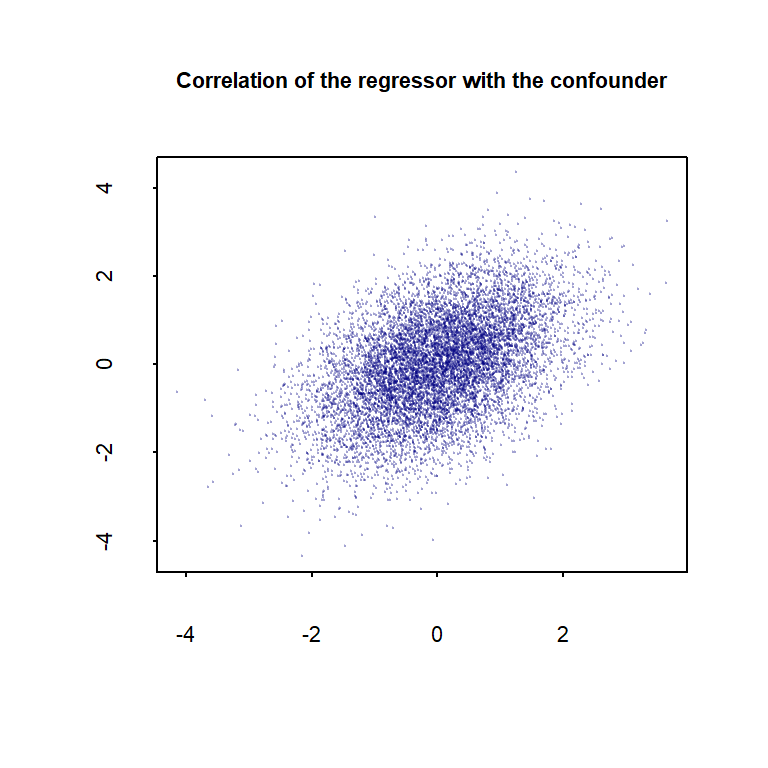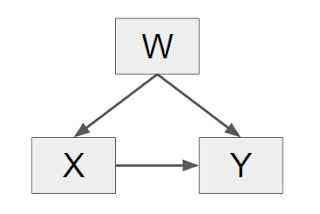### CONFOUNDERS, CONTROLLING, ENDOGENEITY & OMITTED VARIABLE BIAS:

A good deal of the passages below are verbatim copied from the sources quotes, although there are added comments and code.

From the Encyclopedia of Health Economics:

Unobserved Confounder Bias:

At issue here is the presence of confounding variables which serve to mask the true causal effect of $$X$$ on $$Y.$$ The author begins by defining a confounder as a variable that is correlated with both $$X$$ and $$Y.$$ Confounders may be observable and unobservable (denoted $$C_o$$ and $$C_u$$ respectively. In modeling $$Y,$$ if the presence of $$C_u$$ cannot be legitimately ruled out, then $$X$$ is said to be endogenous. Observations on $$C_o$$ can be obtained from the survey data, so its influence can be controlled in estimation of the true causal effect. $$C_u,$$ however, cannot be directly controlled and, if left unaccounted for, will likely cause bias in statistical inference regarding the true causal effect. This happens because estimation methods that ignore the presence of $$C_u$$ will spuriously attribute to $$X$$ observed differences in $$Y$$ that are, in fact, due to $$C_u.$$ The author refers to such bias as unobserved confounder bias (henceforth $$C_u$$-bias) (sometimes called endogeneity bias, hidden selection bias, or omitted variables bias). One can formally characterize $$C_u$$-bias in a useful way. For simplicity of exposition, the author casts the true causal relationship between $$X$$ and $$Y$$ as a linear and writes

$Y = X\beta + C_o\beta_o + C_u\beta_u +e\tag 1$

where $$\beta$$ is the parameter that captures the true causal effect, $$\beta_o$$ and $$\beta_u$$ are parametric coefficients for the confounders, and $$e$$ is the random error term (without loss of generality, it can be assumed that the $$Y$$ intercept is $$0$$). In the naive approach to the estimation of the true causal effect (ignoring the presence of $$C_u$$), the ordinary least squares (OLS) method is applied to

$Y = Xb + C_ob_o+\varepsilon\tag 2$

where the $$b$$’s are parameters and $$\varepsilon$$ is the random error term. The parameter $$b$$ is taken to represent the true causal effect. It can be shown that the OLS will produce an unbiased estimate of $$b.$$ It is also easy to show, however, that

$b=\beta + b_{XC_u}\beta_u\tag 3$

where $$b_{XC_u}$$ is a measure of the correlation between $$C_u$$ and $$X.$$ As it is clear from eqn (3), $$C_u$$-bias in OLS estimation is $$b_{XC_u}\beta_u,$$ which has two salient components: the correlation between the unobserved confounder and the causal variable of interest and the correlation between the unobserved confounder and the outcome. Equation (3) is helpful because it can be used to diagnose potential $$C_u$$-bias. Consider the smoking ($$X$$) and birth weight ($$Y$$) example, in which $$C_u$$ is health mindedness. In this case one would expect that $$b_{XC_u}$$ would be negative and that $$\beta_u$$ would be positive. The net effect of which would be negative $$C_u$$-bias in the estimation of the true causal effect via OLS.

Clearly an approach to estimation is needed that, unlike OLS, does not ignore the presence and potential bias of $$C_u.$$ One such approach exploits the sample variation in a particular type of variable (a so-called instrumental variable - a good explantion can be found on this Quora post) to eliminate bias due to correlation between $$C_u$$ and $$X.$$

Here is a simulation in R:

#Endogenity - Cu is affecting X and Y (hence confounder), but unknown.
set.seed(41)

n <- 1e4 # Number of observations.

Cu <- rnorm(n)  # unobservable confounding variable.
Bu <- 0.3       # Coefficient in the final regression (when calculating the true OLS)
X  <- rnorm(n) + 0.5 * Cu # The unobservable confounding variable affect the regressor of interest. It is correlated approximately 50% with the confounding variable.
cor(X,Cu)
##  0.4561288
plot(Cu,X, pch=20, cex=.2, col=rgb(0,0,.5,.2), xlab="", ylab="", cex.axis=0.7, tck=-.01, main="Correlation of the regressor with the confounder", cex.main=.7)B  <- 0.5      # This is the true coefficient of the regressor of interest.
Co <- rnorm(n) # Co is the observable confounder.
Bo <- 0.3      # It has a .3 coefficient in the real (unknown) OLS.
e  <- rnorm(n) # The error term.
Y  <- B * X + Bo * Co + Bu * Cu + e # The actual generator with Cu indeed influencing Y.

OLStrue <- lm(Y ~ X + Co + Cu) # This is the true causal relationship.
OLSreal_life <- lm(Y ~ X + Co) # This is what we can get without being aware of Cu.

OLStrue$coefficients # The true coefficient in the data generator: ## X ## 0.5076301 This coefficient will be overestimated when Cu is left out (because it is unknown). This is what we will get: OLSreal_life$coefficients #Versus the coefficient we get without Cu:
##         X
## 0.6313806

The idea of endogeneity is simulated “differently” here:

require("MASS")
## Loading required package: MASS
sigma <- matrix(c(1,.5,.5,1),2,2,1)
ex <- mvrnorm(n,rep(0,2),sigma)
x <- ex[,1]
e  <- ex[,2]
Co <- rnorm(n)
y  <- 0.3 * Co + 0.5 * x + e
lm(y ~ Co + x) # The true parameters being 0.3 and 0.5...
##
## Call:
## lm(formula = y ~ Co + x)
##
## Coefficients:
## (Intercept)           Co            x
##   0.0009646    0.2847178    0.9995343

The influence of $$X$$ on the error (unknonw, not calculable, and critically different from the residuals) essentially makes this error term $$e$$ the equivalent to the excluded $$C_u$$ in the simulation above: $$C_u$$ becomes assimilated in the error. In both cases the set up is such that this variable influences both the independent and the dependent variable, as per the definition of confounder.

So, even if the simulation tries to parallel the definition of endogeneity in the linked text:

The following statements allow us to obtain a causal relationship in a regression framework.

$\begin{eqnarray*} y &=& g\left(X\right) + \varepsilon \\ E\left(\varepsilon|X\right) &=& 0 \end{eqnarray*}$

In the expression above, $$y$$ is the outcome vector of interest, $$X$$ is a matrix of covariates, $$\varepsilon$$ is a vector of unobservables, and $$g(X)$$ is a vector-valued function. The statement $$E(\varepsilon \vert X)=0$$ implies that once we account for all the information in the covariates, what we did not include in our model, $$varepsilon,$$ does not give us any information, on average. It also implies that, on average, we can infer the causal relationship of our outcome of interest and our covariates. In other words, it implies that

$\begin{equation*} E\left(y|X\right) = g\left(X\right) \end{equation*}$

The opposite occurs when

$\begin{eqnarray*} y &=& g\left(X\right) + \varepsilon \\ E\left(\varepsilon|X\right) &\neq& 0 \end{eqnarray*}$

The expression $$E(\varepsilon \vert X)≠0$$ implies that it does not suffice to control for the covariates $$X$$ to obtain a causal relationship because the unobservables are not negligible when we incorporate the information of the covariates in our model.

… the inner workings of the simulation are identical. The essential idea, then, is that an exogenous variable, unknown to us, and hence one which cannot be controlled for, influences both $$Y$$ and $$X,$$ which are endogenous. This gives us an error estimating the coefficients - and error that we can expressed as an increase in the non-measurable (in the real world) $$e$$ error, or simly as an omitted unobservable confounder, $$C_u.$$

Sentences that begin with “Controlling for [factors $$X,$$ $$Y,$$ and $$Z$$], …” are reassuring amidst controversial subject matter. But less reassuring is the implicit assumption that $$X,$$ $$Y,$$ and $$Z$$ are indeed the things we call “confounders.” We review the definition of a confounder via the following causal graph:In the above diagram, $$W$$ is a confounder and will distort the percieved causal relationship between $$X$$ and $$Y$$ if unaccounted for. An example from Counterfactuals and Causal Inference: Methods and Principles for Social Research is the effect of educational attainment ($$X$$) on earnings ($$Y$$) where mental ability ($$W$$) is a confounder. The authors remark that the amount of “ability bias” in estimates of educational impact has remained one of the largest causal controversies in the social sciences since the 1970s."

We now review adjustment for confounding via linear models. Open R. Define a sample size that affords us the luxury of ignoring standard errors (without guilt!):

w <- rnorm(n) # This is the confounder, which will affect both X and Y.
x <- .5 * w +          rnorm(n) # The correlation between W and X is 0.5.
y <- .3 * w + .4 * x + rnorm(n) # Here we set up the effect of W and X on the Y variable.

This is essentially identical to the simulation of endogeneity above, except for the fact that no controlable variable $$C_o$$ is included. In this post, the simulation is set up similarly to simulate omitted variable bias.

Note that our confounder $$W$$ is the only variable that is determined from factors outside the system. It is “exogenous,” and the only variable that we can set in any way we want. Both $$X$$ and $$Y$$ depend on $$W,$$ and they are “endogenous” to the system. This is very different than in an experimental design where an artificial grid is created for $$X$$ and those levels prescribed. In that case, $$X$$ would be exogenous too.

Now run the following two regressions in R, noting the very different coefficient estimates for $$X:$$

summary(lm(y ~ x))
##
## Call:
## lm(formula = y ~ x)
##
## Residuals:
##     Min      1Q  Median      3Q     Max
## -3.9362 -0.6998  0.0077  0.6894  3.7460
##
## Coefficients:
##              Estimate Std. Error t value Pr(>|t|)
## (Intercept) -0.001544   0.010380  -0.149    0.882
## x            0.515851   0.009433  54.687   <2e-16 ***
## ---
## Signif. codes:  0 '***' 0.001 '**' 0.01 '*' 0.05 '.' 0.1 ' ' 1
##
## Residual standard error: 1.038 on 9998 degrees of freedom
## Multiple R-squared:  0.2303, Adjusted R-squared:  0.2302
## F-statistic:  2991 on 1 and 9998 DF,  p-value: < 2.2e-16
summary(lm(y ~ x + w))
##
## Call:
## lm(formula = y ~ x + w)
##
## Residuals:
##     Min      1Q  Median      3Q     Max
## -3.9311 -0.6805  0.0010  0.6738  3.7277
##
## Coefficients:
##             Estimate Std. Error t value Pr(>|t|)
## (Intercept) 0.001337   0.010025   0.133    0.894
## x           0.399977   0.010077  39.692   <2e-16 ***
## w           0.299912   0.011152  26.892   <2e-16 ***
## ---
## Signif. codes:  0 '***' 0.001 '**' 0.01 '*' 0.05 '.' 0.1 ' ' 1
##
## Residual standard error: 1.002 on 9997 degrees of freedom
## Multiple R-squared:  0.2822, Adjusted R-squared:  0.282
## F-statistic:  1965 on 2 and 9997 DF,  p-value: < 2.2e-16

The first regression (that ignores $$W$$) incurs upward bias in $$X$$’s coefficient due to the confounder’s positive effects on both $$X$$ and $$Y.$$ The second regression (with $$W$$ included) recovers $$X$$’s true coefficient while increasing the R-squared by a few percentage points. That’s a win-win.